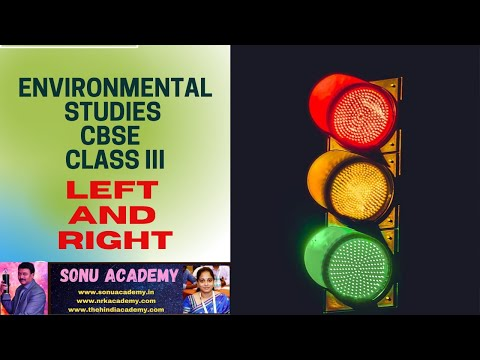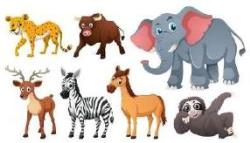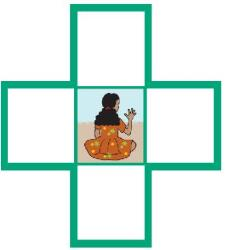Courses

# Test: Right Left Linear Grammar

## 15 Questions MCQ Test Compiler Design | Test: Right Left Linear Grammar

Description
This mock test of Test: Right Left Linear Grammar for Computer Science Engineering (CSE) helps you for every Computer Science Engineering (CSE) entrance exam. This contains 15 Multiple Choice Questions for Computer Science Engineering (CSE) Test: Right Left Linear Grammar (mcq) to study with solutions a complete question bank. The solved questions answers in this Test: Right Left Linear Grammar quiz give you a good mix of easy questions and tough questions. Computer Science Engineering (CSE) students definitely take this Test: Right Left Linear Grammar exercise for a better result in the exam. You can find other Test: Right Left Linear Grammar extra questions, long questions & short questions for Computer Science Engineering (CSE) on EduRev as well by searching above.
QUESTION: 1

### Non-Linear grammar has has two non-terminals on the right-hand side.

Solution:

The above stated grammar is non-linear because it has two non-terminals on the right-hand side.

QUESTION: 2

### S → SS S → λ S → aSb S → bSa which type of grammar is it?

Solution:

Grammar is non-linear because one of the rules (the first one) has two non-terminals on the right-hand side.

QUESTION: 3

### Linear grammar has more than one non-terminal on the right-hand side.

Solution:

Grammar is linear because no rule has more than one non terminal on the right-hand side.

QUESTION: 4

In Right-Linear grammars, all productions have the form: A → xB

Solution:

Right-Linear grammars, following are the form of productions: A → xB or A → x where x is some string of terminals.

QUESTION: 5

S → abS S → a is which grammar

Solution:

grammars in which all of the rules contain only one non-terminal on the left-hand side, and where in every case that non-terminal is the first symbol are called right Linear.

QUESTION: 6

What are the two types of Linear Grammar?

Solution:

Linear grammar is of 2 types Left and Right Linear Grammar

QUESTION: 7

Grammars that can be translated to DFAs:

Solution:
QUESTION: 8

Which Type of Grammar is it?

S → Aa A → Aab | λ

Solution:

In this case they both correspond to the regular expression (ab)*a.

QUESTION: 9

A Regular Grammar is any right-linear or left-linear grammar.

Solution:

As it turns out the languages that can be generated by Regular Grammars is equivalent to those that can be specified by Regular Expressions.

QUESTION: 10

Regular Grammars generate Regular Languages.

Solution:

That’s why they are called regular languages.

QUESTION: 11

Can Left Linear grammar be converted to Right Linear grammar

Solution:

Since right-linear grammars are regular, it follows that left-linear grammars are also regular.

QUESTION: 12

CFG is

Solution:

They are defined by rule A->b where A is non terminal and b is terminal.

QUESTION: 13

The idea of an automation with a stack as auxiliary storage

Solution:

Push Down Automata manipulate the Stack as a part of performing a transition.

QUESTION: 14

Transition of finite automata is

Solution:

Transition of finite automata is Finite Diagram.

QUESTION: 15

A context free language is called ambiguous if

Solution:

When two or more Left and right most derivative occur the grammar turn ambiguous .

Track your progress, build streaks, highlight & save important lessons and more!

### Similar Content### Related tests